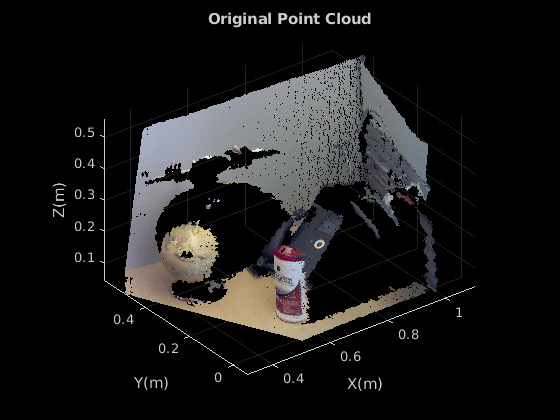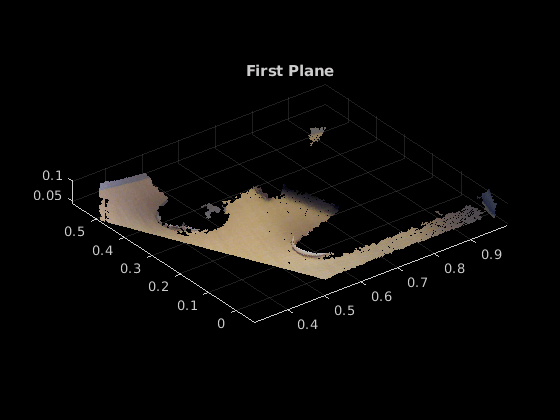Documentation

### This is machine translation

Mouseover text to see original. Click the button below to return to the English version of the page.

## Detect Multiple Planes from Point Cloud

`load('object3d.mat')`

Display and label the point cloud.

```figure pcshow(ptCloud) xlabel('X(m)') ylabel('Y(m)') zlabel('Z(m)') title('Original Point Cloud')```Set the maximum point-to-plane distance (2cm) for plane fitting.

`maxDistance = 0.02;`

Set the normal vector of the plane.

`referenceVector = [0,0,1];`

Set the maximum angular distance to 5 degrees.

`maxAngularDistance = 5;`

Detect the first plane, the table, and extract it from the point cloud.

```[model1,inlierIndices,outlierIndices] = pcfitplane(ptCloud,... maxDistance,referenceVector,maxAngularDistance); plane1 = select(ptCloud,inlierIndices); remainPtCloud = select(ptCloud,outlierIndices);```

Set the region of interest to constrain the search for the second plane, left wall.

```roi = [-inf,inf;0.4,inf;-inf,inf]; sampleIndices = findPointsInROI(remainPtCloud,roi);```

Detect the left wall and extract it from the remaining point cloud.

```[model2,inlierIndices,outlierIndices] = pcfitplane(remainPtCloud,... maxDistance,'SampleIndices',sampleIndices); plane2 = select(remainPtCloud,inlierIndices); remainPtCloud = select(remainPtCloud,outlierIndices);```

Plot the two planes and the remaining points.

```figure pcshow(plane1) title('First Plane')``````figure pcshow(plane2) title('Second Plane')``````figure pcshow(remainPtCloud) title('Remaining Point Cloud')```